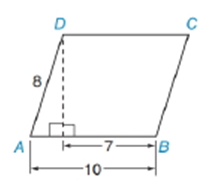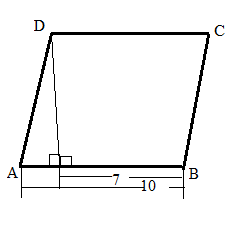Chapter 11.2, Problem 39E### Elementary Geometry for College St...

6th Edition
Daniel C. Alexander + 1 other
ISBN: 9781285195698

#### Solutions

Chapter
Section### Elementary Geometry for College St...

6th Edition
Daniel C. Alexander + 1 other
ISBN: 9781285195698
Textbook Problem
3 views

# In parallelogram ABCD, find to the nearest degree:a) m ∠ A b) m ∠ BTo determine

a) To find:

To find mA by using the following figure,Explanation

Consider the following figure,

In parallelogram ABCD let us take angle at A is α

General formula for cosine ratio and sin ratio is given below,

From the above figure shows that AD = 8 is the hypotenuse value since it is opposite to right angle

To determine

b) To find:

To find mB by using the following figure,### Still sussing out bartleby?

Check out a sample textbook solution.

See a sample solution

#### The Solution to Your Study Problems

Bartleby provides explanations to thousands of textbook problems written by our experts, many with advanced degrees!

Get Started